# Loss Function for Logistic Regression

### Introduction

The first topic that we learn while diving into machine learning is linear Regression, and next to it is logistic Regression. Before getting started on the topic, I recommend you recall the topics of linear Regression.

Let us just quickly revise the logistic regression. Logistic regression is a classification algorithm in supervised learning used to find the probability of a target variable. In simple words, the output of a model is binary(either 0 or 1). Examples of classification problems are online transaction fraud or not fraud, email spam or not, checking whether a person has cancer or not.

All these types of classification lie under logistic regression.

So, what will be the loss function for the above classification problems? In the article below, I will be explaining the loss function used in logistic regression.

### Hypothesis

Firstly, I will define a hypothesis for the linear regression.

h𝝷(x) = 𝝷₀x₀+𝝷₁x₁+𝝷₂x₂+ ... +𝝷ₙxₙ=[𝝷₀+𝝷₁+𝝷₂+ ... +𝝷ₙ]

Where x₀ = 1

Let us Define this hypothesis function of linear Regression as raw model output. In logistic Regression, we have transformed the above hypothesis function. In logistic Regression, we mainly do binary classification based on the output, i.e., 1 or 0. But there are some problems with the hypothesis function of linear Regression; we have to transform it so that its output lies between 0 to 1.  If we look onto a sigmoid function for all values of its output lies between 0 to 1. So for the hypothesis function of Logistic Regression, we use the sigmoid function as shown below.

Sigmoid function: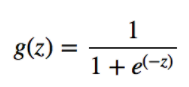Hypothesis function: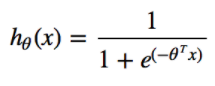Graph of the hypothesis function.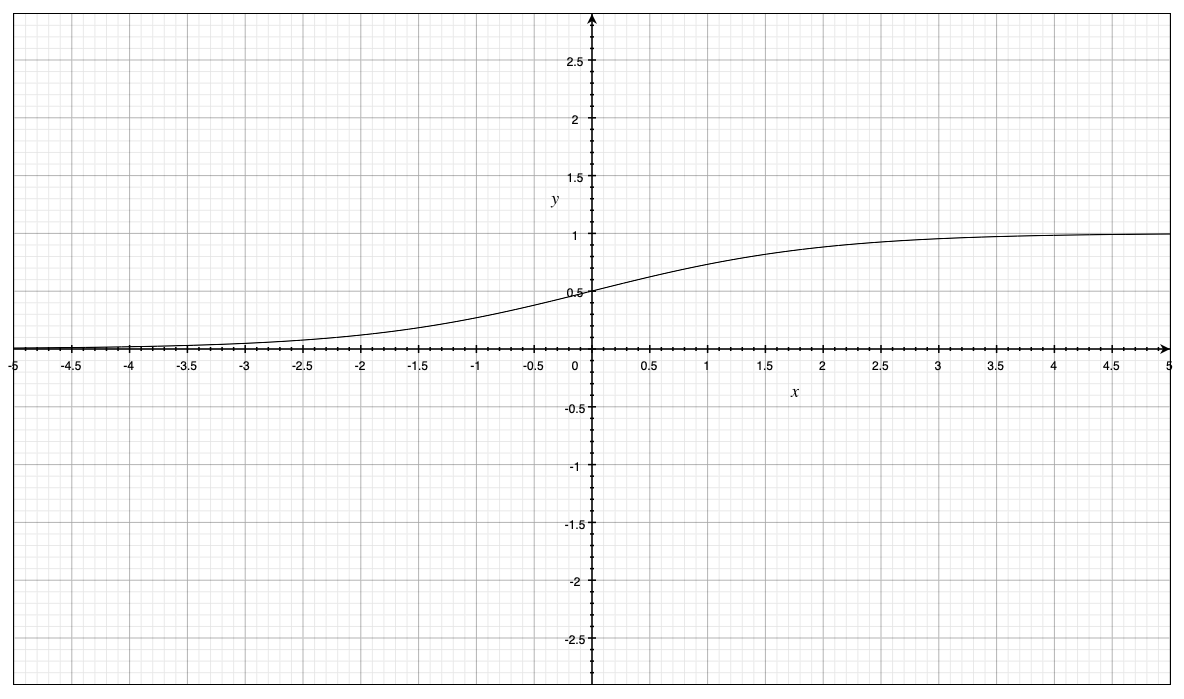In the above graph, we can see that for all values of the hypothesis function lies between 0 to 1.

The output of the above hypothesis function tells the probability of y=1. For given x, parameterized by θ, hypothesis h(x) = P(y = 1|x; θ).

We can describe decision boundary as: Predict 1, if θᵀx ≥ 0 → h(x) ≥ 0.5; Predict 0, if θᵀx < 0 → h(x) < 0.5.

### Cost Function

Let us remember the loss function of Linear Regression; we used Mean Square Error as a loss function as the figure below shows the graph of the loss function of linear Regression.

MSE = (1/n) *∑ (y - Ŷ)²

Here y - Ŷ is the difference between actual and predicted value.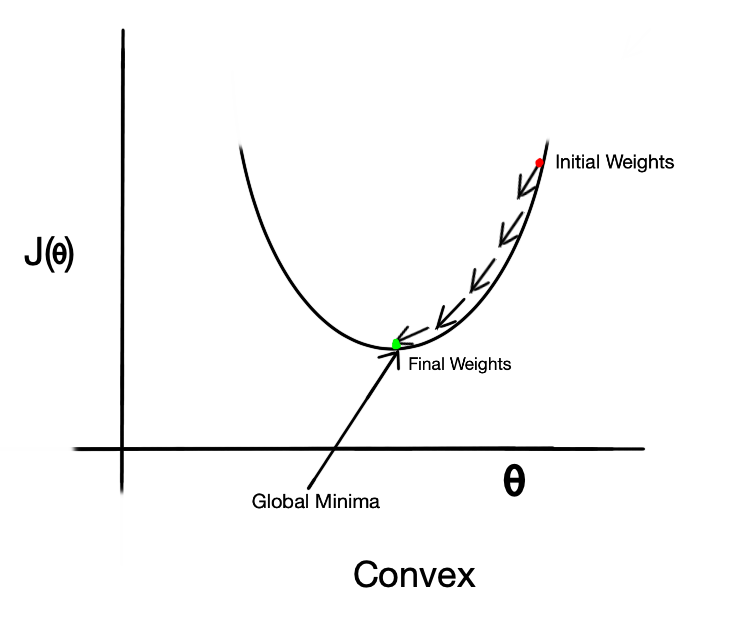When the Gradient Descent Algorithm is applied, all the weights will be adjusted to minimize error.

But in the case of Logistic Regression, we cannot use Mean Squared Error as a loss function because the hypothesis function of logistic is non-linear. Suppose we apply Mean Squared Error as a loss function. In that case, we will get many local minima, and Gradient Descent Algorithm cannot minimize the error as it gets terminated at local minima. The non-linearity introduced by the sigmoid function in the hypothesis causes the non-convex nature of Mean Squared Error with logistic Regression. Relation between weighted parameters and error becomes complex.

Another reason for not using Mean Squared Error in Logistic Regression is that our output lies between 0 - 1. In classification problems, the target value is either 1 or 0. The output of the Logistic Regression is a probability value between 0 to 1. The error (y - p)² will always be between 0-1. Therefore tracking the progress of error value is difficult because storing high precision floating numbers is challenging, and we also cannot round off the digits.

Due to the above reasons, we cannot use Mean Squared Error in logistic Regression.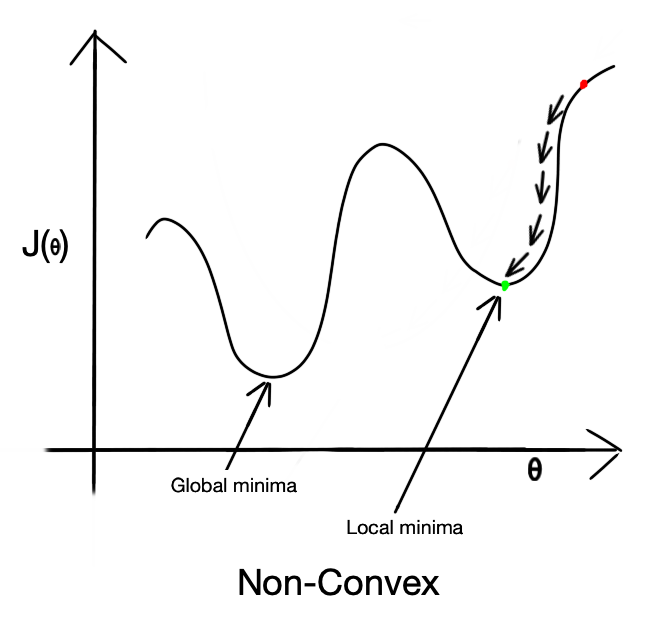Intuitively, we want to assign more punishment when predicting 0 while the actual is 1 and when predicting 1 while the actual is 0. While making loss function, there will be two different conditions, i.e., first when y = 1, and second when y = 0.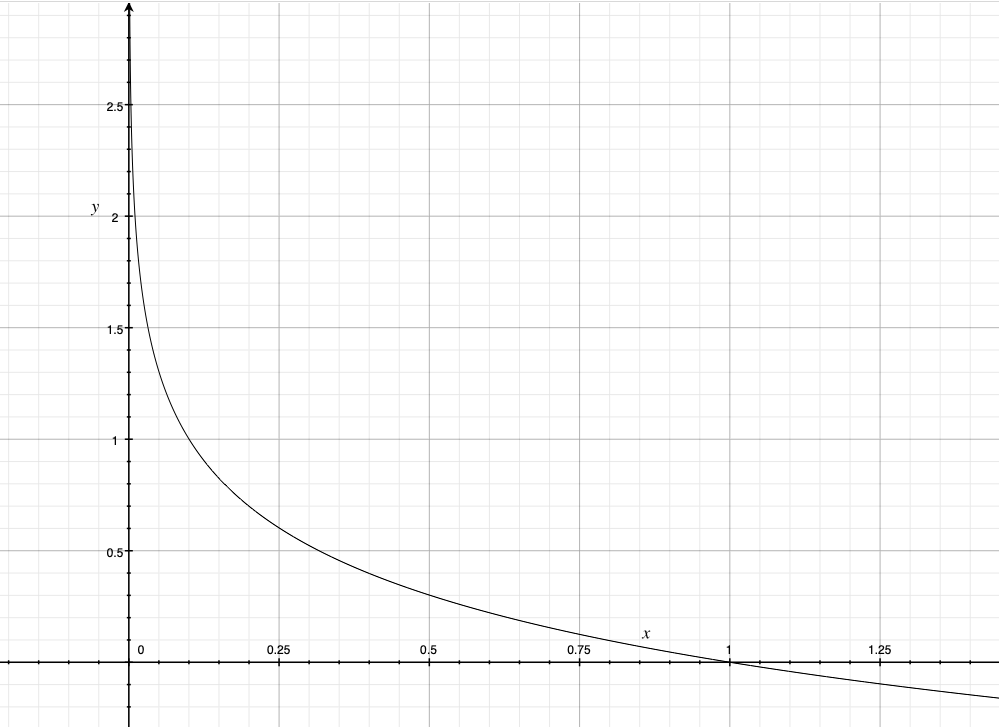The above graph shows the cost function when y = 1. When the prediction is 1, we can see that the cost is 0. When the prediction is 0, the cost is 1. Therefore, a high cost punishes the learning algorithm. (x-axis represents h𝝷(x) predicted value and the y-axis represents cost)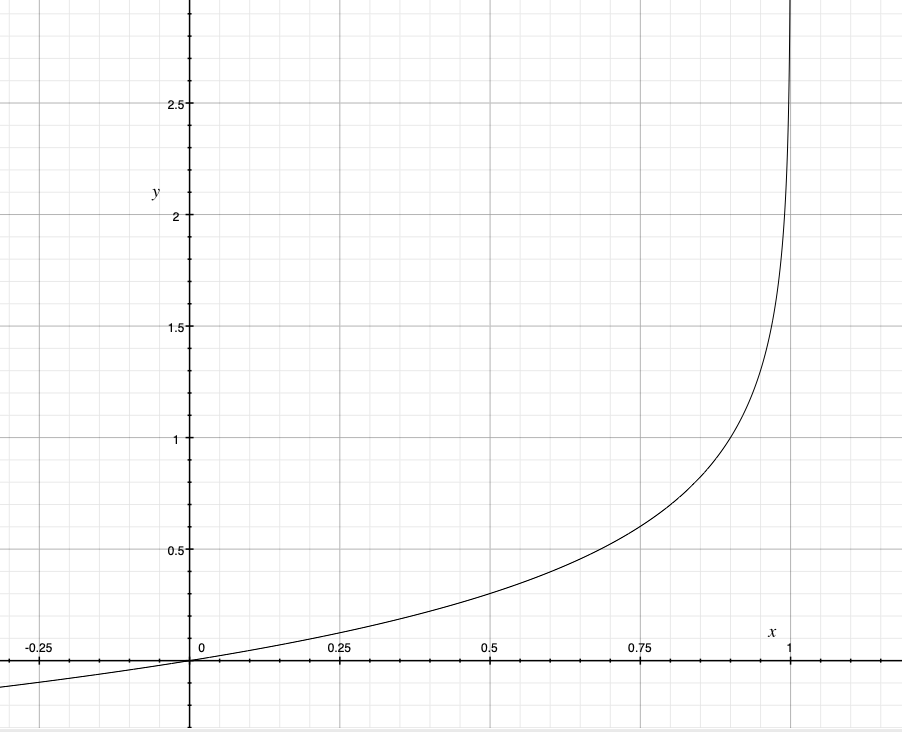The above graph shows the cost function when y = 0. When the prediction is 1, we can see that the cost is 1; therefore, a high cost punishes the learning algorithm. (x-axis represents h𝝷(x) predicted value and the y-axis represents cost)

Therefore the cost function can be defined as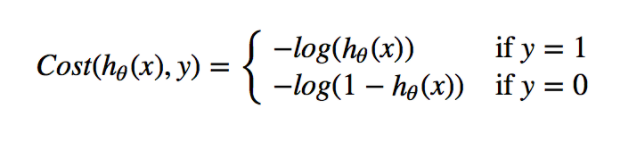Image source

Combining the equation for y=1 and y=0, we get the following equation.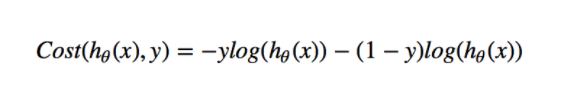Image source

The cost function for the model will be a summation of all the training points.

##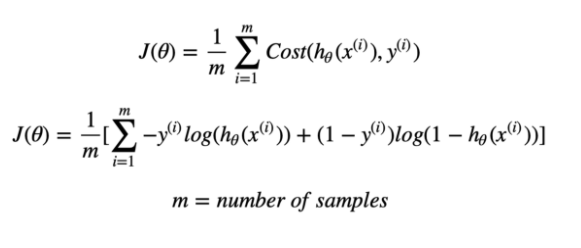Image source

### Implementation

We will see the code for the above-discussed loss function in this part.

## FAQ’s

1. Is it possible to apply a logistic regression algorithm on a 3-class classification problem?
Yes, definitely we can use logistic Regression on a 3-class classification problem using the one vs. all method. Suppose we have three classes A, B, and C, we will train three models, the first model will classify A from B and C, The second model will classify B from A and C, and the third model will classify C from B and A. The model which has a high probability will be considered for classification.

2. Is Logistic Regression mainly used for Regression?
No logistic regression is used for both classification and regression problems. Please don't get confused by its name.

3. Is it possible to design a logistic regression algorithm using a Neural Network Algorithm?
Yes, as the neural network is a universal approximator, a Logistic regression algorithm can be designed using a neural network.

## Key Takeaways

In this article, we have discussed Logistic Regression for loss function. Furthermore, we discussed why the loss function of linear Regression could not be used in logistic Regression. Some important derivations and implementation of the loss functions were covered in this article.

Hello readers, here's a perfect course that will guide you to dive deep into Machine learning.

Happy Coding!